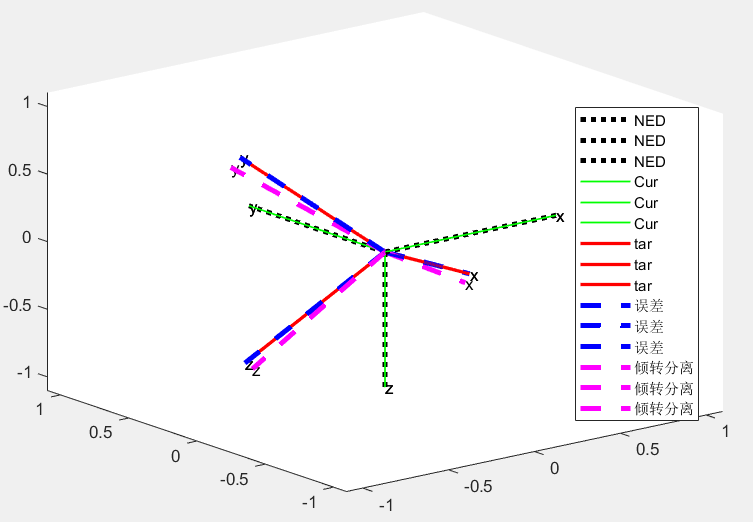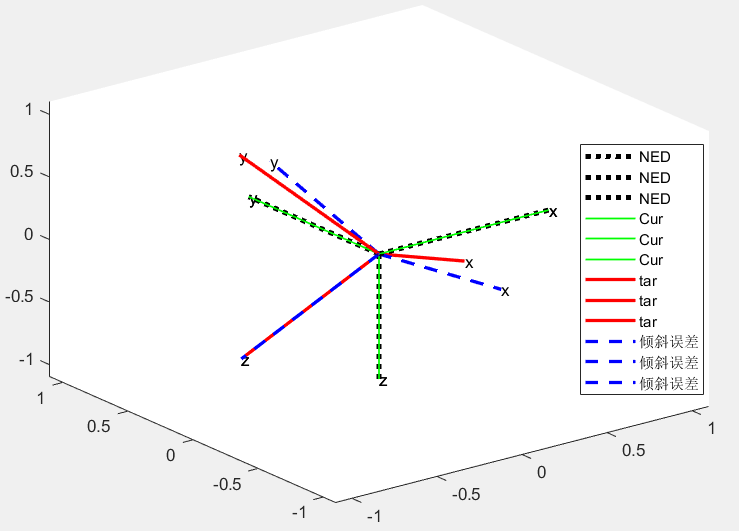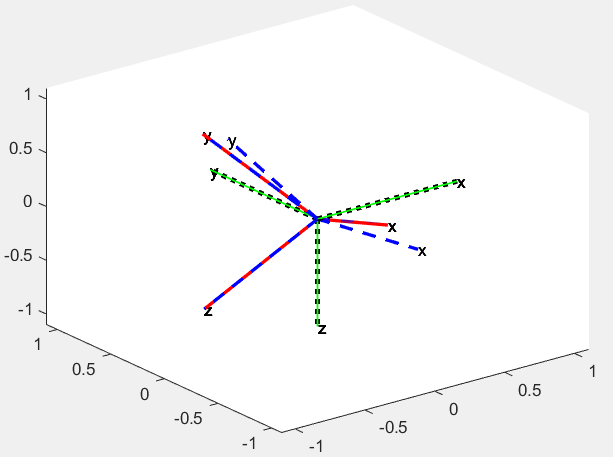# 【飞控】倾转分离 (一)-APM 的倾转分离竟然没有效果？Vector3f rotation;
thrust_vec_correction_quat.to_axis_angle(rotation);
att_diff_angle.x = rotation.x;
att_diff_angle.y = rotation.y;
yaw_vec_correction_quat.to_axis_angle(rotation);
att_diff_angle.z = rotation.z;

1.为什么倾转分离后没对 yaw 做处理（26度以内的yaw）

2.为什么直接抛弃【倾斜误差】的z轴，难道是为了限制yaw？

3.为什么 APM 说是四元数算法，但是过程中各种转旋转矩阵，转轴角，转换次数多了会引入新的误差，倒是PX4，一套四元数用到最后才转轴角，并且每次都会归一化来减少误差。

%NED坐标系 当前机体坐标系 期望机体坐标系 （度）
NED=[0 0 0];
Cur_angle=[0 0 0];
Des_angle=[30 30 25];
%机体坐标系转换为弧度
%在NED下 画出 机体坐标系
plot_rotate_NED([0,0,0],Cur_angle,['g','g','g'],'-',1)
plot_rotate_NED([0,0,0],Des_angle,['r','r','r'],'-',1)

%当前姿态 Ccur->N
%当前姿态的旋转矩阵 取出z轴列（z轴单位向量） Zcur(N)
cur_att_thrust_vec=cur_dcm*[0;0;1];
%期望姿态 Ctar->N
%期望姿态的旋转矩阵 取出z轴列（z轴单位向量） Ztar(N)
des_att_thrust_vec=des_dcm*[0;0;1];

%不使用倾转分离的思路，直接计算 目标姿态-初始姿态=总误差姿态
all_correction_quat = Q_multipli( Q_INV(att_from_quat),att_to_quat );

%%N系下 两个向量的**旋转误差**
thrust_vec_cross=cross(cur_att_thrust_vec,des_att_thrust_vec);
%z轴误差角度 弧度
thrust_vec_dot=acos(dot(cur_att_thrust_vec,des_att_thrust_vec));
thrust_vector_length=norm(thrust_vec_cross);
if( (thrust_vector_length==0) || (thrust_vec_dot==0))
thrust_vec_cross = [0,0,1];
thrust_vec_dot = 0.0;
else
thrust_vec_cross = thrust_vec_cross/thrust_vector_length;
end

%轴角 转 四元数 描述的是 目标转到初始的旋转 所以对应的旋转是 Qtb1->cb 转轴在N系
thrust_vec_correction_quat=axis_angle2Q(thrust_vec_cross,thrust_vec_dot)

%%旋转 Qtb1->cb 转到初始坐标系
thrust_vec_correction_quat=Q_multipli(Q_INV(att_from_quat),Q_multipli(thrust_vec_correction_quat,att_from_quat))

%%画出 初始姿态 加上**倾斜误差** 之后的姿态 结果是 z轴对齐了
plot_rotate_body([0,0,0],Cur_angle,quat2euler(thrust_vec_correction_quat)*57.3,['g','g','g'],'-.',2)
%%画出 初始姿态 加上总误差 之后的姿态 结果是 两个坐标系完全重合
plot_rotate_body([0,0,0],Cur_angle,quat2euler(all_correction_quat)*57.3,['b','b','b'],'--',2)

%Qcb->tb1 * Qn->cb * Qtb->n = Qtb->tb1 (即**旋转误差**)
yaw_vec_correction_quat = Q_multipli(Q_INV(thrust_vec_correction_quat),Q_multipli(Q_INV(att_from_quat),att_to_quat));

all_axis_angle=Q2axis_angle(all_correction_quat);
tilt_axis_angle=Q2axis_angle(thrust_vec_correction_quat);
torsion_axis_angle=Q2axis_angle(yaw_vec_correction_quat);
tilt_torsion_angle=[tilt_axis_angle(1),tilt_axis_angle(2),torsion_axis_angle(3)]*57.3

att_diff_angle_x = tilt_axis_angle(1);
att_diff_angle_y = tilt_axis_angle(2);
att_diff_angle_z = torsion_axis_angle(3);

%att_diff_angle_z 弧度   (0.46弧度 = 26度)
if(att_diff_angle_z > 0.46)
att_diff_angle_z = constrain_float(wrap_PI(att_diff_angle_z), -0.46, 0.46);
yaw_vec_correction_quat=Q_from_axis_angle([0,0,att_diff_angle_z]);
att_to_quat = Q_multipli( att_from_quat,Q_multipli(thrust_vec_correction_quat,yaw_vec_correction_quat));
end